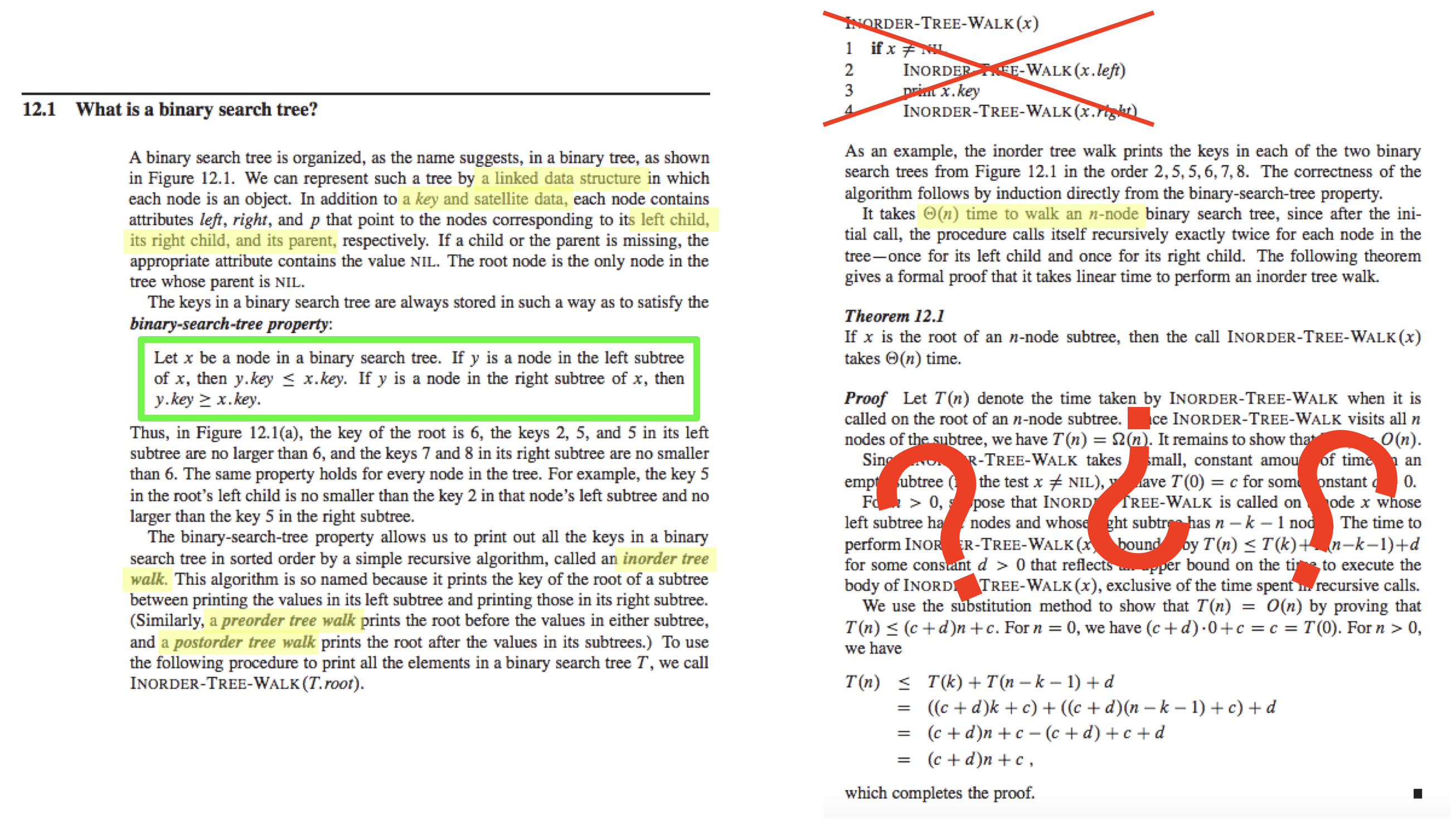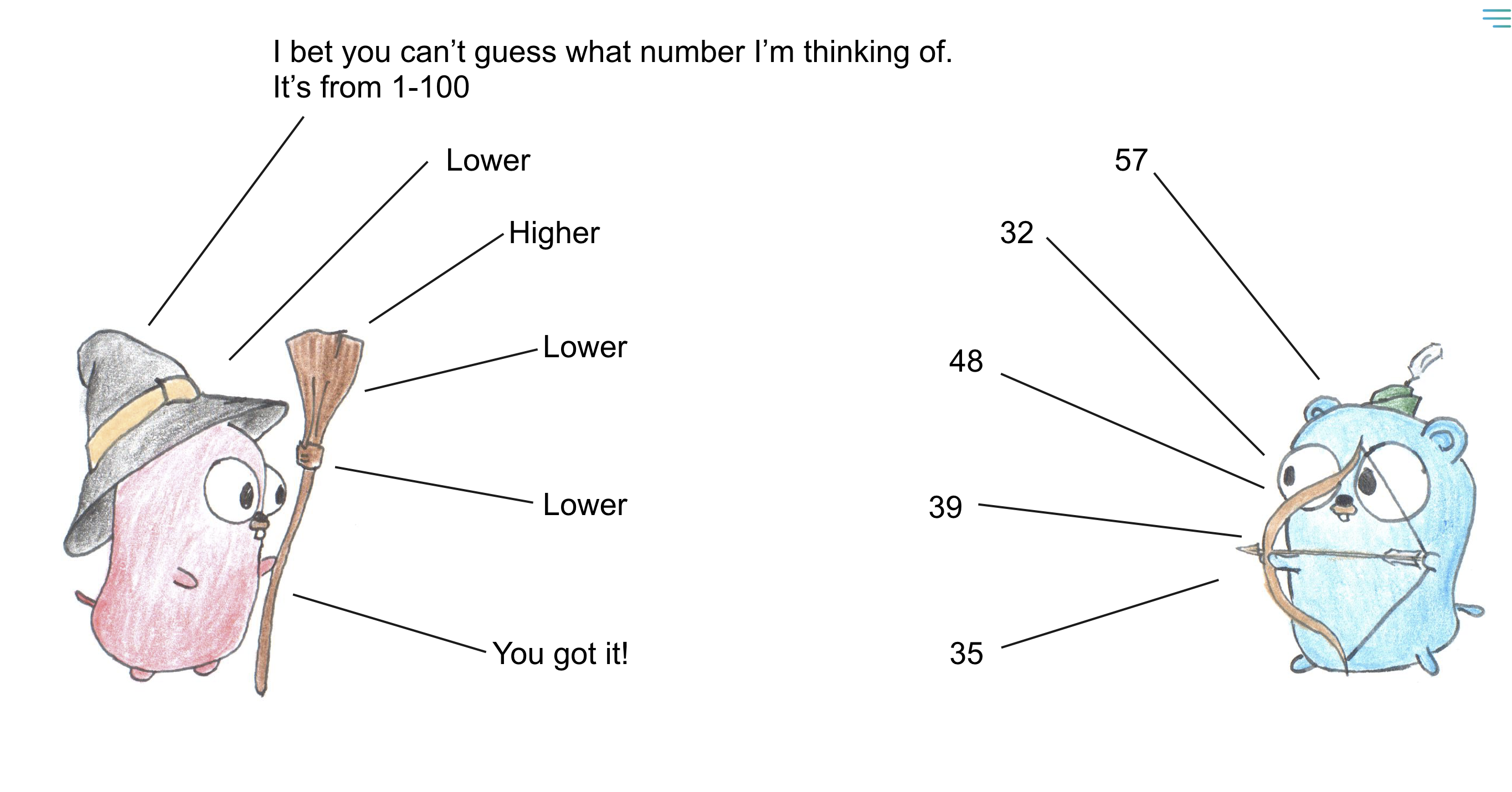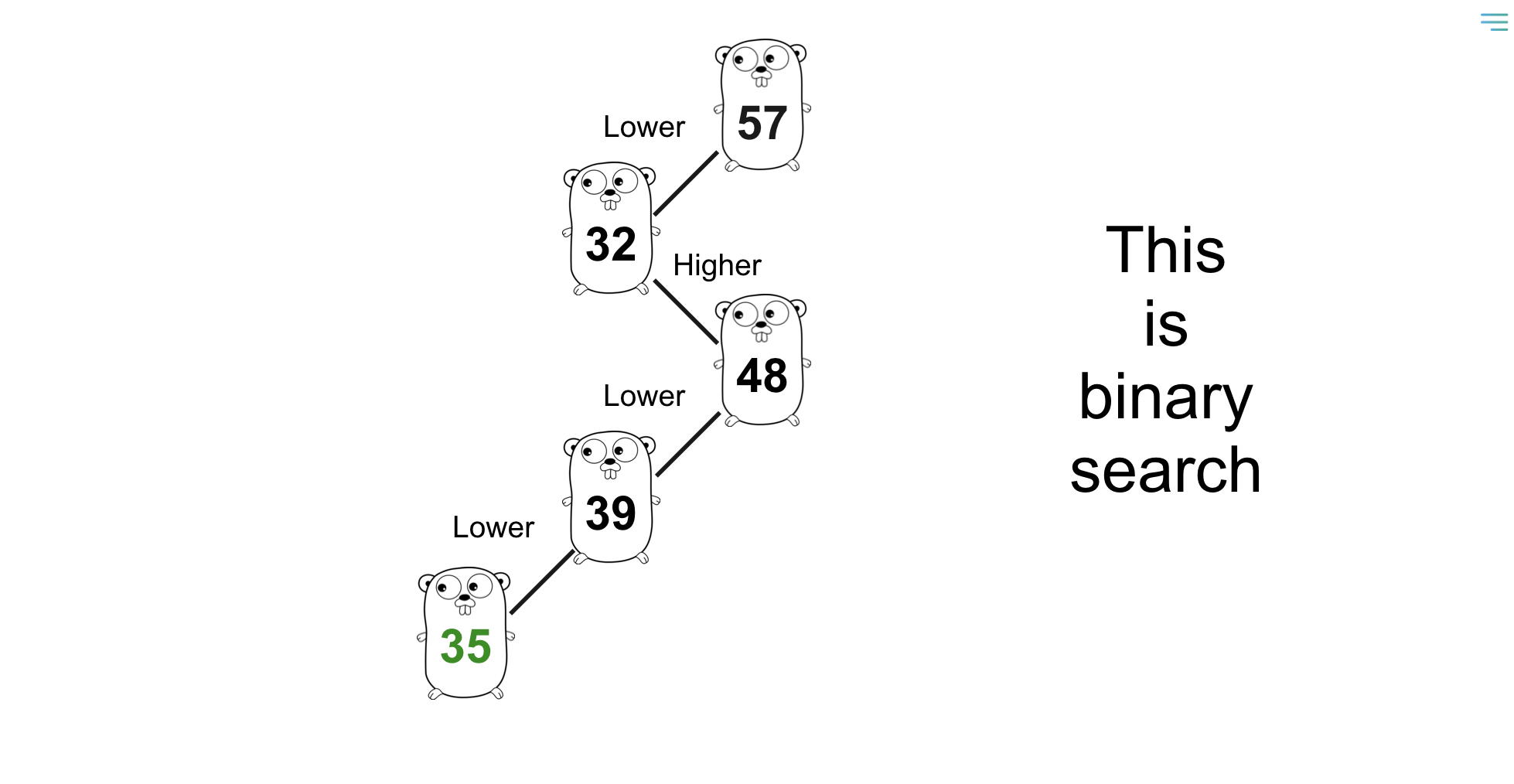# GopherCon 2018 - Demystifying Binary Search Tree Algorithms

Written by Geoffrey Gilmore for the GopherCon Liveblog

Presenter: Kaylyn Gibilterra

Liveblogger: Geoffrey Gilmore

Learning algorithms can be overwhelming and demoralizing, but it doesn't have to be. In this talk, Kaylyn explains binary search tree algorithms in a simple and straightforward manner with code examples in Go.

## Introduction

Kaylyn started writing algorithms for fun over the last year. This might seem strange to you, but it's especially weird for her. She hated her algorithms class in college. Her professor would use complicated jargon and refuse to explain "obvious" concepts. As a result, she only picked up enough knowledge to be able to pass her job interviews and move on with the rest of her life.

Her attitude towards algorithms changed once she started to implement them in Go. Converting algorithms written C or Java into Go was surprisingly easy, and she understood them better afterward than she did back in college.

Kaylyn will explain why that is and show you how to do it with binary search trees, but before we do that: Why exactly is learning algorithms so awful?

## Learning algorithms is horribleThis screenshot is from the binary search tree section of Introduction to Algorithms (a.k.a. CLRS). CLRS is considered to be the "gospel" of algorithms books. To the authors' credit, there hadn't been a great algorithms textbook written before it came out in 1989. However, anyone reading CLRS can tell that it was written by professors whose primary audience was academically minded.

Some examples of this:

• This page references lots of terms that the book defines elsewhere. So you need to know things like

• what satellite data is
• what a linked list is
• what "pre-order" and "post-order" tree traversal mean

You won't know what those things are if you haven't taken notes on every single page in the book.

• If you're anything like Kaylyn, the first thing that you scan the page for is for something that looks like code. However, the only code that's on the page explains one way to walk through a binary search tree - not what a binary search tree actually is.

• The entire bottom quarter of this page is a theorem and a proof. This is probably well-intentioned. Many textbook authors feel that it's essential to prove to you that their statements are true; otherwise, you won't be able to trust them. The irony here is that CLRS is supposed to be an introductory textbook. However, a beginner doesn't need to know all of the exact details of why the algorithm is correct - they'll take your word for it.

• To their credit, they do have a two-sentence area (highlighted in green) explaining what a binary search tree algorithm is. But it's buried in a barely visible sentence that calls it a binary search tree "property", which is confusing terminology for a beginner.

Takeaways:

1. Academic textbook authors aren't necessarily great teachers. The best teachers often don't write textbooks.

2. But most people copy the teaching style/format that the standard textbooks use. Even online resources reference jargon that they think you should know before looking at a binary search tree. In reality, most of this "required knowledge" isn't necessary.

The rest of this talk will cover what a binary search tree is. You'll find it useful if you're new to Go or new to algorithms. If you're neither, it could still be an excellent refresher and something that you can pass on to other people who are curious about these things.

## Guess the Number Game

This is the only thing that you need to know to understand the rest of this talk.This is the "Guess the Number Game", a game that many of you played when you were kids. You'd ask your friends to guess a number within a specific range, like 1-100. Your friend would throw out a number like "57". Their first guess was generally wrong since the range is so broad, but you were allowed to tell them if their guess was higher or lower than the answer. They'd keep guessing new numbers, taking into account the hints that you'd give them, and eventually they'd guess the right answer.This guessing game is pretty much exactly what goes on during binary search. If you understand this guessing game, you also understand the core principal behind binary search tree algorithms. The numbers that they guessed are the numbers in the tree, and the "higher"/"lower" hints tell you the direction that you need to move: either to the right or left child node.

## Rules of Binary Search Trees

1. Each node contains one unique key. You use this to make comparisons between nodes. A key can be any comparable type: a string, integer, etc.
2. Each node has 0, 1, or at most 2 “children” it points to.
3. The keys to the right of any node are greater than that node
4. The keys to the left of any node are less than that node
5. You cannot have duplicate keys

Binary search trees have three major operations:

• Search
• Insert
• Delete

Binary search trees make the above operations fast––that's why they are so popular.The above GIF is an example of searching for the number `39` in the tree.An important point to note is that every node to the right of the node that you are currently looking at is going to be greater than the value of the current node. The nodes to the right of the green line are greater than `57`. All the nodes to the left are smaller than `57`.This property is true for every node in the tree, not just the root node. In the above picture, all the numbers to the right of the green line are greater than `32`, all the numbers to the left of the red line are smaller than `32`.

So, now that we know the fundamentals, let's start writing some code.

``````type Node struct {
Key   int
Left  *Node
Right *Node
}``````

The fundamental structure that we are going to use is a `struct`. If you are new to `struct`s, they are essentially just a collection of fields. The three fields that you need are `Key` (used for comparisons with other nodes), and the `Left` and `Right` child nodes.

When you want to define a `Node`, you can do it using a struct literal:

``tree := &Node{Key: 6}``

This creates a `Node` with a `Key` value `6`. You might be wondering where the `Left` and `Right` fields are. Nothing is stopping you from providing all the fields:

``````tree := &Node{
Key:   6,
Left:  nil,
Right: nil,
}``````

However, you are allowed to specify only a subset of the `struct`'s fields if you a provide field names (e.g., `Key` in this case).

You can also instantiate a `Node` without named fields:

``tree := &Node{6, nil, nil}``

In this case: the first argument is `Key`, the second argument is `Left`, and the third argument is `Right`.

After you instantiate your `Node`, you can access your struct's fields using dot notation like this:

``````tree := &Node{6, nil, nil}
fmt.Println(tree.Key)``````

So, let's write the algorithm for `Search`:

``````func (n *Node) Search(key int) bool {
// This is our base case. If n == nil, `key`
// doesn't exist in our binary search tree.
if n == nil {
return false
}

if n.Key < key { // move right
return n.Right.Search(key)
} else if n.Key > key { // move left
return n.Left.Search(key)
}

// n.Key == key, we found it!
return true
}``````

## InsertThe above GIF is an example of inserting `81` into the tree. Insert is very similar to search. We want to find where `81` should go inside the tree, so we walk the tree using the same rules that we used in `search`, and insert `81` once we find an empty spot.

``````func (n *Node) Insert(key int) {
if n.Key < key {
if n.Right == nil { // we found an empty spot, done!
n.Right = &Node{Key: key}
} else { // look right
n.Right.Insert(key)
}
} else if n.Key > key {
if n.Left == nil { // we found an empty spot, done!
n.Left = &Node{Key: key}
} else { // look left
n.Left.Insert(key)
}
}
// n.Key == key, don't need to do anything
}``````

If you haven't seen the `(n *Node)` syntax before, it's known as a pointer receiver.

## DeleteThe above GIF is an example of deleting `78` from the tree. We search for `78` like we've done before, and in this case, we're able to just "snip" `78` from the tree by directly connecting `85` as the right child of `57`.

``````func (n *Node) Delete(key int) *Node {
// search for `key`
if n.Key < key {
n.Right = n.Right.Delete(key)
} else if n.Key > key {
n.Left = n.Left.Delete(key)
// n.Key == `key`
} else {
if n.Left == nil { // just point to opposite node
return n.Right
} else if n.Right == nil { // just point to opposite node
return n.Left
}

// if `n` has two children, you need to
// find the next highest number that
// should go in `n`'s position so that
// the BST stays correct
min := n.Right.Min()

// we only update `n`'s key with min
// instead of replacing n with the min
// node so n's immediate children aren't orphaned
n.Key = min
n.Right = n.Right.Delete(min)
}
return n
}``````

## Min valueIf you move to the left over and over, you get the smallest number (`24` in this case).

``````func (n *Node) Min() int {
if n.Left == nil {
return n.Key
}
return n.Left.Min()
}``````

## Max value``````func (n *Node) Max() int {
if n.Right == nil {
return n.Key
}
return n.Right.Max()
}``````

If you move to the right over and over, you get the largest number (`96` in this case).

## Testing

Since we've written the code for each of the primary functions of binary search trees, let's actually test our code! Testing was the most fun part of the process for Kaylyn: testing in Go is much more straightforward than a lot of other languages like Python and C.

``````// You only need to import one library!
import "testing"

// This is called a test table. It's a way to easily
// specify tests while avoiding boilerplate.
// See https://github.com/golang/go/wiki/TableDrivenTests
var tests = []struct {
input  int
output bool
}{
{6, true},
{16, false},
{3, true},
}

func TestSearch(t *testing.T) {
//     6
//    /
//   3
tree := &Node{Key: 6, Left: &Node{Key: 3}}

for i, test := range tests {
if res := tree.Search(test.input); res != test.output {
t.Errorf("%d: got %v, expected %v", i, res, test.output)
}
}

}``````

All you have to do to run this is:

``> go test``

Go runs your tests and will print a nicely formatted result that tells you if your tests passed, error messages from your test failures, and how long your tests took.

## Benchmarking

But wait––there's more! Go also makes it easy to do some very simple benchmarking. This is all the code that you need:

``````import "testing"

func BenchmarkSearch(b *testing.B) {
tree := &Node{Key: 6}

for i := 0; i < b.N; i++ {
tree.Search(6)
}
}``````

`b.N` will run `tree.Search()` over and over again until it observes a stable execution time for `tree.Search()`.

You can run this test with just the following command:

``> go test -bench=``

and your output will look like this:

``````goos: darwin
goarch: amd64
pkg: github.com/kgibilterra/alGOrithms/bst
BenchmarkSearch-4       1000000000               2.84 ns/op
PASS
ok      github.com/kgibilterra/alGOrithms/bst   3.141s``````

The line that you want to pay attention to is this:

``BenchmarkSearch-4       1000000000               2.84 ns/op``

This line tells you the speed of the function you have. In this case `test.Search()` took ~2.84 nanoseconds to execute.

Since it's easy to run benchmarks, you can start running some experiments such as:

• What happens if I use a much larger / deeper tree?
• What happens if I change the key that I am searching for?

Kaylyn found it particularly helpful for things like understanding the performance characteristics between maps and slices. It's nice to get quick, tactile feedback about things that you read about online.

## Binary Search Tree Terminology

The last thing that we're going to take a look at is some binary search tree terminology. If you decide to learn more about binary search trees on your own, it'll be useful for you know to about these terms:

Tree height: Number of edges on the longest path from the root to the base. This determines the speed of your algorithm.This tree has a height of `5`.

Node depth: Number of edges from the root to that node

`48` is `2` edges away from the root.Full binary tree: Each node has exactly 0 or 2 childrenComplete binary tree: The tree is entirely filled, except for the bottom row, which can be filled from left to rightAn unbalanced treeImagine that you're searching for `47` in this tree. Do you see how that takes `7` steps while searching for `24` only takes three? This problem becomes more exacerbated the more "unbalanced" the tree is. The way to fix it is to "balance" the tree:

A balanced tree:This tree contains the same nodes as the unbalanced tree, but searches on balanced trees are faster (on average) than on unbalanced trees.